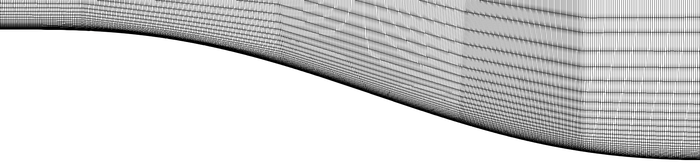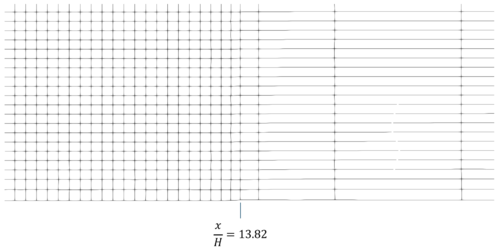# Computational Details

This section provides details of the computational approach used for the simulation of the present flow problem. Firstly, the main numerical features of the code MIGALE are given. Then, information about the computational grid is provided. Finally, the statistical quantities and their computation are explained.

## Computational approach

The CFD code MIGALE can solve both compressible and incompressible flow problems and implements different flow models ranging from the Euler to the RANS equations. The solver uses the Discontinuous Galerkin (DG) method for the spatial discretization of the governing equations, here the compressible Navier-Stokes equations. The discontinuous nature of the numerical solution requires introducing a special treatment of the inviscid interface flux and of the viscous flux. For the former it is common practice to use suitably defined numerical flux functions, which ensure conservation and account for wave propagation. To this purpose, in the compressible case, the exact Riemann solver of Gottlieb et al. (1988) is mainly used. For the latter, the 2nd-scheme of Bassi and Rebay (BR2) is employed, see Bassi et al. (1997). The devised method uses hierarchical and orthonormal polynomial basis functions defined in the physical (mesh) space and relies for the time integration on, primarily, high-order Rosenbrock linearly-implicit schemes, see Bassi et al. (2015). The Jacobian matrix of the spatial discretization is computed analytically and linear systems are solved using preconditioned GMRES methods from the PETSc library (Portable Extensible Toolkit for Scientific Computations). For production runs time integration is coupled with time-step adaptation strategies able to improve the robustness and the efficiency in terms of time-to-solution of simulations, see Noventa et al. (2020).

For the present computation the fourth-order DG spatial discretization is combined with the fifth-order eight stages Rosenbrock-type scheme of Di Marzo (1993).

## Spatial and temporal resolution, grids

The computational domain extends in streamwise direction from $x/H=-12.7$to $x/H=24.0$and has a spanwise width equal to $\Delta z/H=3$. Top boundary is located at normal coordinate $y/H=180$, where $y/H=0$is the normal coordinate of the solid wall downstream the rounded step. The grid is made of roughly 15 million elements with quadratic edges, resulting in more than 300 million Degrees of Freedom (DoF) per equation. Fig. 3 and Fig. 4 show details of the grid above the rounded step (lateral view) and the wall region (top view), respectively. In Fig. 4 and Fig. 5 the coarse mesh region created downstream of the rounded step, i.e., for $x/H>13.82$, is clearly visible. The mesh coarsening is applied to reduce the solution gradients, thus mitigating spurious perturbations possibly originating at the outlet boundary.Figure 3: Detail of the computational grid above the rounded step (lateral view)Figure 4: Detail of the computational grid above the wall region downstream the rounded step (top view)Figure 5: Detail of the computational grid above the wall region (lateral view)

For the temporal integration, a fifth order linearly implicit Rosenbrock scheme is applied with an adaptive time stepping technique. The resulting average time step is roughly $\Delta t\approx 1/285\,CTU$, where $CTU$is the convective time unit defined with respect to the reference velocity and the step height. In Tab. 1 are reported the wall space and time resolution at the checkpoint ($x_{ckp}/H=-3.5$). Here, in order to account for the high-order nature of the numerical schemes employed, the wall resolution is obtained as

$\Delta x^{+}={{\dfrac {1}{\sqrt[{3}]{N_{DoF}}}}{\dfrac {\Delta x}{\delta _{\nu }}}},\qquad \Delta t^{+}={{\dfrac {1}{q}}{\dfrac {\Delta t\,u_{\tau }}{\delta _{\nu }}}}$,

where $N_{DoF}$is the number of Degrees of Freedom per equation and $q$the time integration order (here $N_{DoF}=20$and $q=5$).

The flow has been simulated for $40\,CTU$with a DG polynomial approximation of degree 1. After that, the polynomial degree has been increased to 3 and the simulation has been continued for $50\,CTU$before gathering statistics for additional $75\,CTU$.

 ${\Delta x^{+}}$${\Delta y_{1}^{+}}$${\Delta z^{+}}$${\Delta t^{+}}$${17.8}$${1.30}$${18.6}$${0.10}$Table 1: Near-wall space and time resolution at the checkpoint ($x_{ckp}/H=-3.5$)

## Computation of statistical quantities

Within code MIGALE turbulence statistics are collected on the run (see Appendix B of the list of desirable quantities (PDF)) in a set of points within each mesh element. The points location is the same as that of a nodal multi-dimensional Lagrangian basis obtained by the tensor product of a one-dimensional Lagrange basis built on a set of evenly spaced points at edges. The number of points on each edge is set to $k+1$to be able to properly represent a $k$-degree polynomial function. This choice results in $(k+1)^{3}$points for each element.

The statistics collected during the production run are those of the list of baseline quantities for compressible codes reported in Tab. 4 of the list of desirable quantities (PDF). Starting from this list, the set of statistics reported in Tab.1 of the same document is computed in a single postprocessing step exploiting simple algebraic manipulations and spatial derivations. In particular, spatial derivatives are computed analytically within each mesh element starting from a least square approximation of degree $k+1$over the set of points.